Friday, October 22, 2021
Home > ICSE Solutions for Class 10 Maths > S Chand ICSE Maths Solutions for Class 10 Chapter 4 Linear Inequations Exercise 4

# S Chand ICSE Maths Solutions for Class 10 Chapter 4 Linear Inequations Exercise 4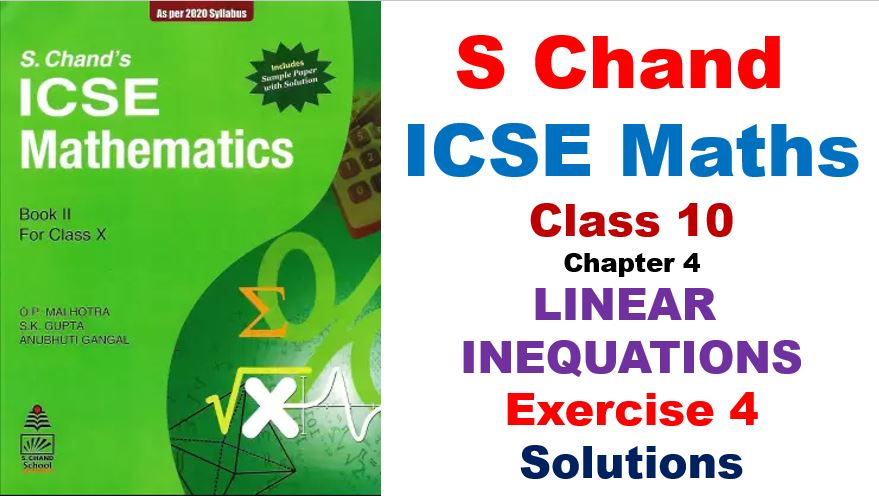# S Chand ICSE Maths Solutions for Class 10 Chapter 4 Linear Inequations in One Variables

Hi students, Welcome to Amans Maths Blogs (AMB). In this post, you will get S Chand ICSE Maths Solutions for Class 10 Chapter 4 Linear Inequations in One Variable Exercise 4. This is the fourth chapter ‘Linear Inequations in One Variables‘ included in ICSE 2021 Class 10 Maths syllabus.

## S Chand ICSE Maths Solutions for Class 10 Chapter 4 Exercise 4

### S Chand ICSE Solutions for Class 10 Maths Exercise 4: Ques No 1

On a bargain counter, the shopkeeper puts labels on various goods showing their prices, Rs. P, where P is real numbers. Write a mathematical sentences for each of the following labels

(a) more than Rs. 7.50

(b) not less than Rs. 10

(c) not more than Rs. 22

(d) less than Rs. 11

S Chand ICSE Maths Solutions:

Since the price on label is Rs P, then

(a) more than Rs. 7.50 ⇒ P > 7.50

(b) not less than Rs. 10 ⇒ P ≮ 10 or P ≥ 10

(c) not more than Rs. 22 ⇒ P ≯  22 or P ≤ 10

(d) less than Rs. 11 ⇒ P < 11

### S Chand ICSE Solutions for Class 10 Maths Exercise 4: Ques No 2

You are given the following numbers : -2.6, 5.1, -3, 0.4, 1.2, -3.1, 4.7

Fill in the blanks

(a) A = {x : x ≥ -3 } = {……..}

(b) B = {x : x ≤ 1 } = {……..}

S Chand ICSE Maths Solutions:

You are given the following numbers : -2.6, 5.1, -3, 0.4, 1.2, -3.1, 4.7

It mean, you are given a replacement set as U = {-3.1, -3, -2.6, 0.4, 1.2, 4.7, 5.1}.

(a) Solution of A = {x : x ≥ -3 } = {-3, -2.6, 0.4, 1.2, 4.7, 5.1}

(b) Solution of B = {x : x ≤ 1 } = {-3.1, -3, -2.6, 0.4}

### S Chand ICSE Solutions for Class 10 Maths Exercise 4: Ques No 3

If the replacement set is {-2, -1, +1, +2, +4, +5, +9}, what is the solution set of each of the following mathematical senetences.

(a) x + 3/2 > 5/2

(b) x – 4 = -3

(c) 2x – 5 ≥ 10

(d) 3y/2 ≤ 5/2

(e) 4x2 = 16.

S Chand ICSE Maths Solutions:

Since the replacement set is {-2, -1, +1, +2, +4, +5, +9}, then

(a) Solution set of x + 3/2 > 5/2 ⇒ x > 5/2 – 3/2 ⇒ x > 1 is {+2, +4, +5, +9}.

(b) Solution set of x – 4 = -3 ⇒ x = -3 + 4 ⇒ x = 1 is {+1}.

(c) Solution set of 2x – 5 ≥ 10 ⇒ 2x ≥ 15 ⇒ x ≥ 7.5 is {+9}.

(d) Solution set of 3y/2 ≤ 5/2 ⇒ 3y ≤ 5 ⇒ y ≤ 5/3 ⇒ y ≤ 1.66 is {-2, -1, +1}.

(e) Solution set of 4x2 = 16 ⇒ x2 = 4 ⇒ x = +2 or -2 is {-2, +2}.

### S Chand ICSE Solutions for Class 10 Maths Exercise 4: Ques No 4

List the solution set of 30 – 4(2x – 1) < 30, given that x is a positive integer.

S Chand ICSE Maths Solutions:

Since the replacement set is a positive integer, then

Solution set of

30 – 4(2x – 1) < 30

⇒ -4(2x – 1) < 30 – 30

⇒ -4(2x – 1) < 0

⇒ (2x – 1) > 0

⇒ x > 1/2

⇒ x > 0.5

is x = {1, 2, 3, 4, 5,…}

### S Chand ICSE Solutions for Class 10 Maths Exercise 4: Ques No 5

If the replacement set is {0, 1, 2, 3, 4, 5, 6, 7, 8, 9, 10}, what is the solution set of each of the following mathematical senetences.

(a) x + 4/3 = 7/3

(b) 2x + 1 < 3

(c) x – 6 > 10 – 6

(d) x + 5 = 20

(e) 2x + 3 ≥ 17

S Chand ICSE Maths Solutions:

Since the replacement set is {0, 1, 2, 3, 4, 5, 6, 7, 8, 9, 10}, then

(a) Solution set of x + 4/3 = 7/3 ⇒ x = 3/3 ⇒ x = 1 is {1}.

(b) Solution set of 2x + 1 < 3 ⇒ 2x < 2 ⇒ x < 1 is {0}.

(c) Solution set of x – 6 > 10 – 6 ⇒ x > 10 is φ.

(d) Solution set of x + 5 = 20 ⇒ x = 15 is φ.

(e) Solution set of 2x + 3 ≥ 17 ⇒ 2x ≥ 14 ⇒ x ≥ 7 is {7, 8, 9}.

### S Chand ICSE Solutions for Class 10 Maths Exercise 4: Ques No 6

(a) x ∈ {2, 4, 6, 9} and y ∈ {4, 6, 18, 27, 54}. Form all ordered pairs (x, y) such that x is a factor of y and x < y.

(b) Find the truth set of the inequality x > y + 2, where (x, y) ∈ {(1, 2), (2, 3), (5, 1), (7, 3), (5, 6)}

S Chand ICSE Maths Solutions:

(a) x ∈ {2, 4, 6, 9} and y ∈ {4, 6, 18, 27, 54}.

(x, y) = x is a factor of y and x < y.

2 is a factor of 4, 6, 18, 54 and 2 < 4, 2 < 6, 2 < 18, 2 < 54, then (x, y) forms the ordered pairs as (2, 4), (2, 6), (2, 18), (2, 54).

4 is a factor of 4 but 4 = 4, then (4, 4) is not in (x, y).

6 is a factor of 18, 54 and 6 < 18, 6 < 54, then (x, y) forms the ordered pairs as (6, 18), (6, 54).

9 is a factor of 18, 27, 54 and 9 < 18, 9 < 27, 9 < 54 then (x, y) forms the ordered pairs as (9, 18), (9, 27), (9, 54).

Thus, the solution set of (x, y) is {(2, 4), (2, 6), (2, 18), (2, 54), (6, 18), (6, 54), (9, 18), (9, 27), (9, 54)}.

(b) Given inequality is x > y + 2. It means x is greater than y + 2.

In {(1, 2), (2, 3), (5, 1), (7, 3), (5, 6)}, we see that (5, 1) and (7, 3) are two pairs which satisfy the given inequality.

Thus, the solution set of (x, y) is {(5, 1), (7, 3)}.

### S Chand ICSE Solutions for Class 10 Maths Exercise 4: Ques No 7

Find out the truth set of the following open sentences replacement sets are given

(i) 5/x > 7 ; {1, 2}

(ii) 5/x > 2 ; {1, 2, 3, 4, 5, 6}

(iii) x2 = 9 ; {-3, -2, -1, 1, 2, 3}

(iv) x + 1/x = 2 ; {0, 1, 2, 3}

(v) 3x2 < 2x ; {-4, -3, -2, -1, 0, 1, 2, 3, 4}

(vi) 2(x – 3) < 1 ; {1, 2, 3, 4, 5, 6, 7, 8, 9, 10}

S Chand ICSE Maths Solutions:

(i) Given replacement set is {1, 2}.

Now given inequality is 5/x > 7 ⇒ x < 5/7 ⇒ x < 0.71

Thus, the solution set of the given inequality is x ∈ φ.

(ii) Given replacement set is {1, 2, 3, 4, 5, 6}.

Now given inequality is 5/x > 2 ⇒ x < 5/2 ⇒ x < 2.5

Thus, the solution set of the given inequality is x ∈ {1, 2}.

(iii) Given replacement set is {-3, -2, -1, 1, 2, 3}.

Now given equation is x2 = 9 ⇒ x = 3 or -3.

Thus, the solution set of the given equation is x ∈ {-3, 3}.

(iv) Given replacement set is {0, 1, 2, 3}.

Now given equation is x + 1/x = 2 ⇒ (x2 + 1) = 2x ⇒ (x2 -2x + 1) = 0 ⇒ (x – 1)2 = 0 ⇒ (x – 1) = 0 ⇒ x = 1.

Thus, the solution set of the given equation is x ∈ {1}.

(v) Given replacement set is {-4, -3, -2, -1, 0, 1, 2, 3, 4}.

Now given inequality is 3x2 < 2x ⇒ 3x2 – 2x < 0 ⇒ x(3x – 2) < 0 ⇒ 0 < x < 2/3 ⇒ 0 < x < 0.66

Thus, the solution set of the given inequality is x ∈ φ.

(vi) Given replacement set is {1, 2, 3, 4, 5, 6, 7, 8, 9, 10}.

Now given inequality is 2(x – 3) < 1 ⇒ x – 3 < 0.5 ⇒ x < 3.5.

Thus, the solution set of the given inequality is x ∈ {1, 2, 3}.

### S Chand ICSE Solutions for Class 10 Maths Exercise 4: Ques No 8

Statement: The sum of the lengths of any two sides of a triangle is always greater than the length of its third side.

Let x, x+1, x+2 be the lengths of the three sides of a triangle.

(i) Write down the three inequations in x, each of which represents the given statement.

(ii) List the set of possible values of x which satisfy all the three inequations obtained in your answer to part (i) above, given that x is an integer.

S Chand ICSE Maths Solutions:

The lengths of the three sides of a triangle are x, x+1, x+2.

(i)

Since the sum of the lengths of any two sides of a triangle is always greater than the length of its third side, we have three inequality as below.

x + (x + 1) > (x + 2) ⇒ 2x + 1 > x + 2 ⇒ x > 1   …(1)

x + (x + 2) > (x + 1) ⇒ 2x + 2 > x + 1 ⇒ x > -1  …(2)

(x + 1) + (x + 2) > x ⇒ 2x + 3 > x ⇒ x > -3        …(3)

On combining (1), (2), (3), we get x > 1.

(ii) Since replacement set of x is an integer, means x ∈ {…, -3, -2, -1, 0, 1, 2, 3, …}.

The solution set of x > 1 is x ∈ { 2, 3, 4, …}.

### S Chand ICSE Solutions for Class 10 Maths Exercise 4: Ques No 9

(a) If x + 10 = y + 14, then x > y

(b) |-4| – 4 = 8

(c) If 10 – x > 3, then x < 7

(d) If p = q + 2, then p > q

(e) If a and b are two negative integers such that a < b, then 1/a < 1/b

(f) 3 ∈ {x : 3x – 2 ≥ 5

S Chand ICSE Maths Solutions:

(a) x + 10 = y + 14 ⇒ x – y = 14 – 10 ⇒ x – y = 4 ⇒ x – y > 0 ⇒ x > y.

Thus, the given statement “If x + 10 = y + 14, then x > y” is TRUE.

(b) |-4| – 4 = 4 – 4 = 0

Thus, the given statement “|-4| – 4 = 8” is FALSE.

(c) 10 – x > 3 ⇒ 10 – 3 > x ⇒ 7 > x ⇒ x > 7

Thus, the given statement “If 10 – x > 3, then x < 7” is TRUE.

(d) p = q + 2 ⇒ p – q = 2 ⇒ p – q > 0 ⇒ p > q

Thus, the given statement “If p = q + 2, then p > q” is TRUE.

(e) a < b ⇒ 1/a > 1/b

Thus, the given statement “If a and b are two negative integers such that a < b, then 1/a < 1/b” is FALSE.

(f) 3x – 2 ≥ 5 ⇒ 3x ≥ 7 ⇒ x ≥ 7/3 ⇒ x ≥ 2.3

Thus, the given statement “3 ∈ {x : 3x – 2 ≥ 5}” is TRUE.

### S Chand ICSE Solutions for Class 10 Maths Exercise 4: Ques No 10

Find the solution of the inequation 2 ≤ 2p – 3 ≤ 5. Hence graph the solution set on the number line.

S Chand ICSE Maths Solutions:

2 ≤ 2p – 3 ≤ 5 ⇒ 5 ≤ 2p ≤ 8 ⇒ 5/2 ≤ p ≤ 4 ⇒ 2.5 ≤ p ≤ 4

The solution on the number line is as below.### S Chand ICSE Solutions for Class 10 Maths Exercise 4: Ques No 11

If x is a negative integer, find the solution set of 2/3 + (x + 1)/3 > 0.

S Chand ICSE Maths Solutions:

2/3 + (x + 1)/3 > 0(x + 1)/3 > -2/3 ⇒ x + 1 > -2 ⇒ x > -3 …(1)

Since the replacement set of x is negative integer, it means x ∈ {…, -5, -4, -3, -2, -1}.

From (1),  x ∈ {-2, -1}.

### S Chand ICSE Solutions for Class 10 Maths Exercise 4: Ques No 12

Write open mathematical sentences, using x for the variable whose graphs would be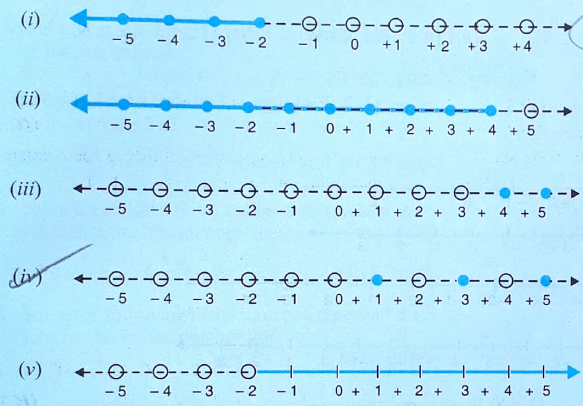S Chand ICSE Maths Solutions:

(i)

From the graph, we see that the shaded arrow is left of -2 (included) and all the real numbers are taken up to -2.

Thus, required mathematical sentence is {x : x ≤ -2 and x ∈ R}.

(ii)

From the graph, we see that the shaded arrow is left of 4 (included) and all the real numbers are taken up to 4.

Thus, required mathematical sentence is {x : x ≤ 4 and x ∈ R}.

(iii)

From the graph, we see that the solid circles are at 4 and 5 and only natural numbers are taken.

Thus, required mathematical sentence is {x : 4 x ≤ 5 and x ∈ N}.

(iv)

From the graph, we see that the solid circles are at 1, 3 and 5 and only natural numbers are taken.

Thus, required mathematical sentence is {x : 1 x ≤ 5 and x ∈ N}.

(v)

From the graph, we see that the shaded arrow is right of -2 (excluded) and all the real numbers are ahead of -2.

Thus, required mathematical sentence is {x : x > -2 and x ∈ R}.

### S Chand ICSE Solutions for Class 10 Maths Exercise 4: Ques No 13

(i) If 2 – x < 0, then x > 2.

(ii) The graph of the inequations y ≤ 2x includes the origin.

S Chand ICSE Maths Solutions:

(i) 2 – x < 0 ⇒ 2 < x.

Thus, the given statement is TRUE.

(ii) On putting x = 0 and y = 0 in y ≤ 2x, we get that 0 = 0, which is true.

Thus, the given statement is TRUE.

### S Chand ICSE Solutions for Class 10 Maths Exercise 4: Ques No 14

If 25 – 4x ≤ 16, then find

(i) the smallest value of x when x is real number.

(ii) the smallest values of x when x is an integer.

S Chand ICSE Maths Solutions:

25 – 4x ≤ 16 ⇒ 25 – 16 ≤ 4x ⇒ 9 ≤ 4x ⇒ x ≥ 9/4.

(i) Thus, the smallest value of x is 9/4 = 2 1/4.

(ii) The smallest integer greater than x ≥ 9/4 is 3.

### S Chand ICSE Solutions for Class 10 Maths Exercise 4: Ques No 15

Given x ∈ {1, 2, 3, 4, 5, 6, 7, 9}, find the values of x for which -3 < 2x – 1< x + 4

S Chand ICSE Maths Solutions:

Given replacement set is x ∈ {1, 2, 3, 4, 5, 6, 7, 9}.

Given inequation is -3 < 2x – 1 < x + 4.

On solving -3 < 2x – 1 ⇒ 2x > -3 + 1 ⇒ 2x > -2 ⇒ x > -1 … (1)

On solving 2x – 1 < x + 4 ⇒ 2x – x < 1 + 4 ⇒ x < 5   …(2)

From (1) and (2), we get -1 < x < 5.

Thus, the solution set of the given inequation is x ∈ {1, 2, 3, 4}.

### S Chand ICSE Solutions for Class 10 Maths Exercise 4: Ques No 16

Solve the inequality 2x – 10 < 3x – 15

S Chand ICSE Maths Solutions:

Given inequality is 2x – 10 < 3x – 15

⇒ 2x – 3x < 10 – 15

⇒ -x < -5

⇒ x > 5

### S Chand ICSE Solutions for Class 10 Maths Exercise 4: Ques No 17

Solve the inequation 3 – 2x ≥ x – 12, given that x ∈ N.

S Chand ICSE Maths Solutions:

Given inequation is 3 – 2x ≥ x – 12

⇒ -x – 2x ≥ -3 – 12

⇒ -3x ≥ -15

⇒ x ≤ 5

Since the replacement of x is natural number, then the solution set of the given inequation is

x ∈ {1, 2, 3, 4, 5}.

### S Chand ICSE Solutions for Class 10 Maths Exercise 4: Ques No 18

x ∈ {real numbers} and -1 < 3 – 2x ≤ 7, evaluate x and represent it on number line.

S Chand ICSE Maths Solutions:

Given inequation is -1 < 3 – 2x ≤ 7

From -1 < 3 – 2x,

⇒ -1 < 3 – 2x ⇒ -1 – 3 < – 2x ⇒ -4 < -2x ⇒ 2x < 4 ⇒ x < 2 …(1)

and 3 – 2x ≤ 7

⇒ 3 – 7 ≤ 2x ⇒ -4 ≤ 2x ⇒ -2 ≤ x …(2)

On combining (1) and (2), we get -2 ≤ x < 2.

The graph of this inequation is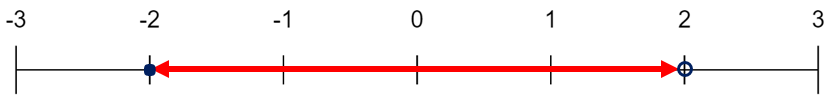### S Chand ICSE Solutions for Class 10 Maths Exercise 4: Ques No 19

Find the range of values of x which satisfies and graph these values of x on number line.S Chand ICSE Maths Solutions:

Given inequation is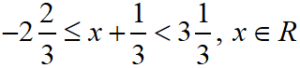On simplifying, we getNow the graph of these values of x is as below.### S Chand ICSE Solutions for Class 10 Maths Exercise 4: Ques No 20

Solution and graph the solution set of

(a)(b)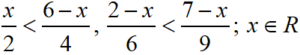S Chand ICSE Maths Solutions:

(a) Given inequation isOn solving first inequation, we get 6 ≥ 2 – x ⇒ x ≥ 2 – 6 ⇒ x ≥ -4 … (1)

On solving second inequation, we get x/3 + 2 < 3 ⇒ x/3 < 3 – 2 ⇒ x/3 < 1 ⇒ x < 3  … (2)

On combining (1) and (2), we get -4 ≤ x < 3.

The graph of this inequation is(b) Given inequation isOn solving first inequation, we get

x/2 < (6 – x)/4 ⇒ 2x < 6 – x ⇒ 3x < 6 ⇒ x < 2… (1)

On solving second inequation, we get

(2 – x)/6 < (7 – x)/9 ⇒ 3(2 – x) < 2(7 – x) ⇒ 6 – 3x < 14 – 2x ⇒ x > -8  … (2)

On combining (1) and (2), we get -8 < x < 2.

The graph of this inequation is### S Chand ICSE Solutions for Class 10 Maths Exercise 4: Ques No 21

Find the range of values of x which satisfy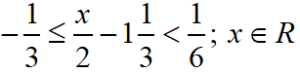Graph these values of x on real number line.

S Chand ICSE Maths Solutions:

Given inequation isOn simplifying,we getGraph of these values of x on number line is as below.### S Chand ICSE Solutions for Class 10 Maths Exercise 4: Ques No 22

Write down the range of real values of x for which the inequation x > 3 and -2 ≤ x < 5 are both true.

S Chand ICSE Maths Solutions:

Graphing the given inequations x > 3 and -2 ≤ x < 5 on same number line, we get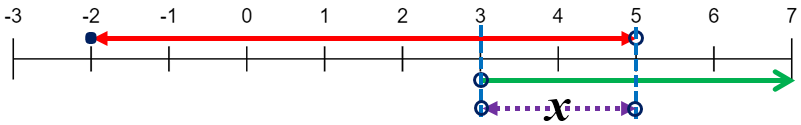Thus, we get 3 < x < 5.

### S Chand ICSE Solutions for Class 10 Maths Exercise 4: Ques No 23

Solve and graph the solution set of 3x – 4 > 11 or 5 – 2x ≥ 7;  x ∈ R.

S Chand ICSE Maths Solutions:

On solving the first inequation, we get 3x – 4 > 11 ⇒ 3x > 15 ⇒ x > 5 …(1)

On solving the second inequation, we get 5 – 2x ≥ 7 ⇒ – 2x ≥ 2 ⇒ x ≤ 1 …(2)

On combining (1) and (2), we get x ≤ 1 or x > 5.

AMBiPi
error: Content is protected !!